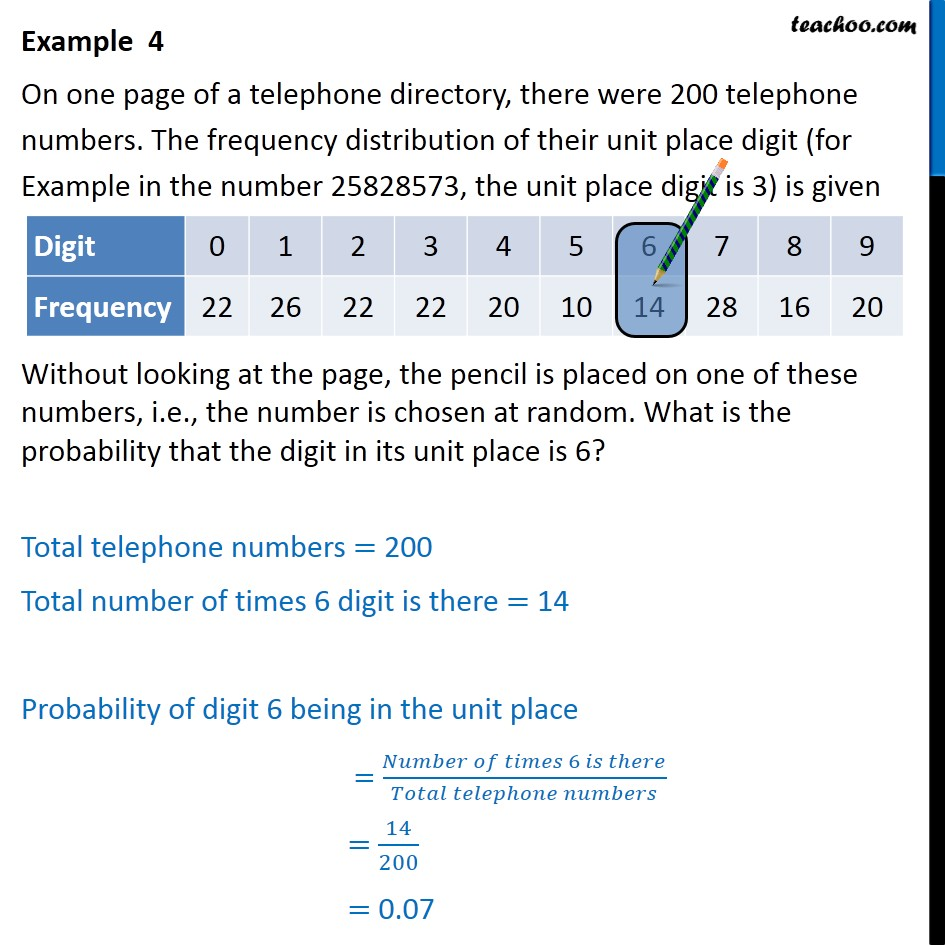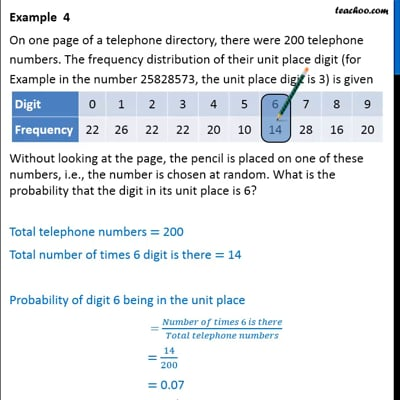Examples

Chapter 15 Class 9 Probability (Term 2)
Serial order wiseThis video is only available for Teachoo black users

### Transcript

Example 4 On one page of a telephone directory, there were 200 telephone numbers. The frequency distribution of their unit place digit (for Example in the number 25828573, the unit place digit is 3) is given Without looking at the page, the pencil is placed on one of these numbers, i.e., the number is chosen at random. What is the probability that the digit in its unit place is 6? Total telephone numbers = 200 Total number of times 6 digit is there = 14 Probability of digit 6 being in the unit place = 14/200 = 0.07